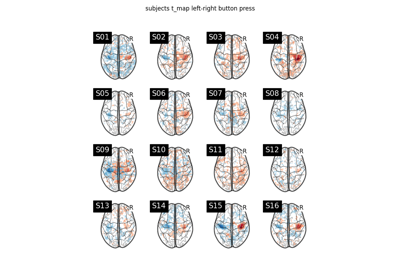Note

This page is a reference documentation. It only explains the function signature, and not how to use it. Please refer to the user guide for the big picture.

# 8.12.16.3. nilearn.glm.second_level.non_parametric_inference¶

nilearn.glm.second_level.non_parametric_inference(second_level_input, confounds=None, design_matrix=None, second_level_contrast=None, mask=None, smoothing_fwhm=None, model_intercept=True, n_perm=10000, two_sided_test=False, random_state=None, n_jobs=1, verbose=0)[source]

Generate p-values corresponding to the contrasts provided based on permutation testing. This function reuses the ‘permuted_ols’ function Nilearn.

Parameters
second_level_inputpandas DataFrame or list of Niimg-like objects.

If a pandas DataFrame, then they have to contain subject_label, map_name and effects_map_path. It can contain multiple maps that would be selected during contrast estimation with the argument first_level_contrast of the compute_contrast function. The DataFrame will be sorted based on the subject_label column to avoid order inconsistencies when extracting the maps. So the rows of the automatically computed design matrix, if not provided, will correspond to the sorted subject_label column.

If list of Niimg-like objects then this is taken literally as Y for the model fit and design_matrix must be provided.

confoundspandas DataFrame, optional

Must contain a subject_label column. All other columns are considered as confounds and included in the model. If design_matrix is provided then this argument is ignored. The resulting second level design matrix uses the same column names as in the given DataFrame for confounds. At least two columns are expected, “subject_label” and at least one confound.

design_matrixpandas DataFrame, optional

Design matrix to fit the GLM. The number of rows in the design matrix must agree with the number of maps derived from second_level_input. Ensure that the order of maps given by a second_level_input list of Niimgs matches the order of the rows in the design matrix.

second_level_contraststr or array of shape (n_col), optional

Where `n_col` is the number of columns of the design matrix. The default (None) is accepted if the design matrix has a single column, in which case the only possible contrast array((1)) is applied; when the design matrix has multiple columns, an error is raised.

Mask to be used on data. If an instance of masker is passed, then its mask will be used. If no mask is given, it will be computed automatically by a MultiNiftiMasker with default parameters. Automatic mask computation assumes first level imgs have already been masked.

smoothing_fwhmfloat, optional

If smoothing_fwhm is not None, it gives the size in millimeters of the spatial smoothing to apply to the signal.

model_interceptbool, optional

If True, a constant column is added to the confounding variates unless the tested variate is already the intercept. Default=True.

n_permint, optional

Number of permutations to perform. Permutations are costly but the more are performed, the more precision one gets in the p-values estimation. Default=10000.

two_sided_testboolean, optional

If True, performs an unsigned t-test. Both positive and negative effects are considered; the null hypothesis is that the effect is zero. If False, only positive effects are considered as relevant. The null hypothesis is that the effect is zero or negative. Default=False.

random_stateint or None, optional

Seed for random number generator, to have the same permutations in each computing units.

n_jobsint, optional

Number of parallel workers. If -1 is provided, all CPUs are used. A negative number indicates that all the CPUs except (abs(n_jobs) - 1) ones will be used. Default=1.

verboseint, optional

Verbosity level (0 means no message). Default=0.

Returns
neg_log_corrected_pvals_imgNifti1Image

The image which contains negative logarithm of the corrected p-values.

## 8.12.16.3.1. Examples using `nilearn.glm.second_level.non_parametric_inference`¶